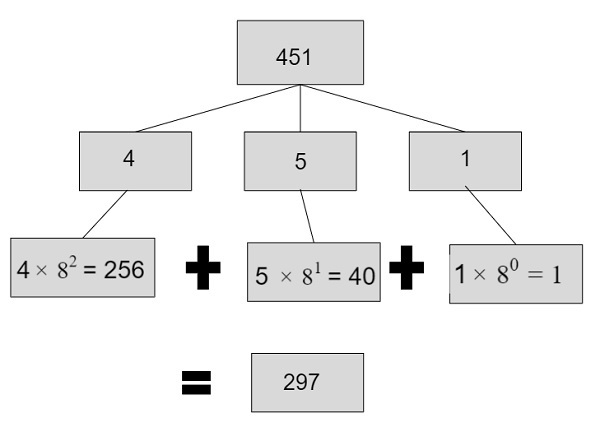# Program for octal to decimal conversion in C++

Given with an octal number as an input, the task is to convert the given octal number into a decimal number.

Decimal numbers in computer is represented with base 10 and octal number is represented with base 8 starting from the digit 0 till 7 whereas decimal numbers can be any numeric digit starting from 0 – 9.

To convert an octal number into a decimal number follow these steps −

• We will extract digits starting from right to left through a remainder and then multiply it with the power starting from 0 and will be increased by 1 till the (number of digits) – 1
• Since we need to do conversions from octal to binary the base of power will be 8 as octal have base 8.
• Multiply the digits of given inputs with base and power and store the results
• Add all the multiplied values to obtain the final result which will be a decimal number.

Given below is the pictorial representation of converting an octal number into a decimal number.## Example

Input-: 451
1 will be converted to a decimal number by -: 1 X 8^0 = 1
5 will be converted to a decimal number by -: 5 X 8^1 = 40
4 will be converted to a decimal number by -: 4 X 8^2 = 256
Output-: total = 0 + 40 + 256 = 10

## Algorithm

Start
Step 1-> declare function to convert octal to decimal
int convert(int num)
set int temp = num
set int val = 0
set int base = 1
Set int count = temp
Loop While (count)
Set int digit = count % 10
Set count = count / 10
Set val += digit * base
Set base = base * 8
End
return val
step 2-> In main()
set int num = 45
Call convert(num)
Stop

## Example

Live Demo

#include <iostream>
using namespace std;
//convert octal to decimal
int convert(int num) {
int temp = num;
int val = 0;
int base = 1;
int count = temp;
while (count) {
int digit = count % 10;
count = count / 10;
val += digit * base;
base = base * 8;
}
return val;
}
int main() {
int num = 45;
cout <<"after conversion value is "<<convert(num);
}

## Output

IF WE RUN THE ABOVE CODE IT WILL GENERATE FOLLOWING OUTPUT

after conversion value is 37